# Physical Chemistry Topics

The best high school and college tutors are just a click away, 24×7! Pick a subject, ask a question, and get a detailed, handwritten solution personalized for you in minutes. We cover Math, Physics, Chemistry & Biology.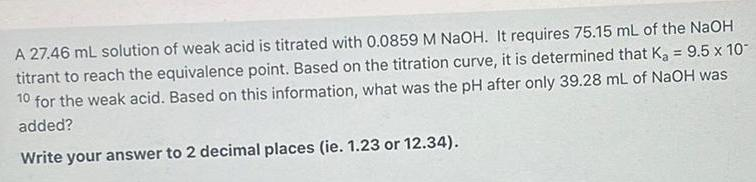Physical Chemistry
Solutions
A 27.46 mL solution of weak acid is titrated with 0.0859 M NaOH. It requires 75.15 mL of the NaOH titrant to reach the equivalence point. Based on the titration curve, it is determined that K₂ = 9.5 x 10- 10 for the weak acid. Based on this information, what was the pH after only 39.28 mL of NaOH was added? Write your answer to 2 decimal places (ie. 1.23 or 12.34).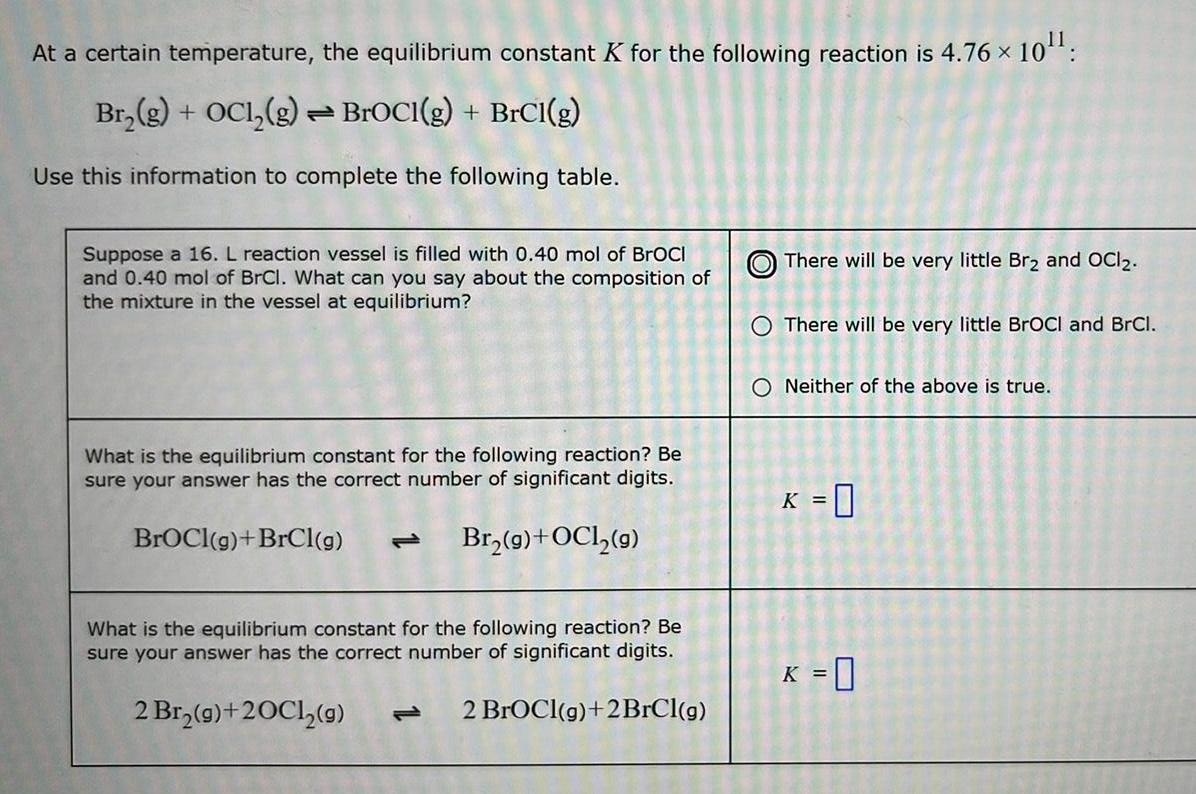Physical Chemistry
General
At a certain temperature, the equilibrium constant K for the following reaction is 4.76 × × 10¹¹: Br₂(g) + OC1₂(g) → BrOCI(g) + BrCl(g) Use this information to complete the following table. Suppose a 16. L reaction vessel is filled with 0.40 mol of BrOCI and 0.40 mol of BrCl. What can you say about the composition of the mixture in the vessel at equilibrium? What is the equilibrium constant for the following reaction? Be sure your answer has the correct number of significant digits. BrOCI(g) +BrCl(g) Br₂(g) + OCl₂(g) What is the equilibrium constant for the following reaction? Be sure your answer has the correct number of significant digits. 2 Br₂(g) +20C1₂(g) 2 BrOCI(g) +2BrCl(g) There will be very little Br₂ and OCI2. O There will be very little BrOCI and BrCl. O Neither of the above is true. K = 0 K = 0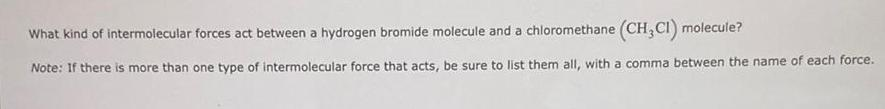Physical Chemistry
Chemical Bonding
What kind of intermolecular forces act between a hydrogen bromide molecule and a chloromethane (CH,CI) molecule? Note: If there is more than one type of intermolecular force that acts, be sure to list them all, with a comma between the name of each force.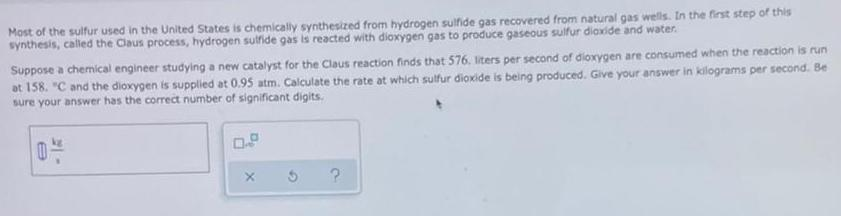Physical Chemistry
Gaseous and liquid states
Most of the sulfur used in the United States is chemically synthesized from hydrogen sulfide gas recovered from natural gas wells. In the first step of this synthesis, called the Claus process, hydrogen sulfide gas is reacted with dioxygen gas to produce gaseous sulfur dioxide and water. Suppose a chemical engineer studying a new catalyst for the Claus reaction finds that 576. liters per second of dioxygen are consumed when the reaction is run at 158. "C and the dioxygen is supplied at 0.95 atm. Calculate the rate at which sulfur dioxide is being produced. Give your answer in kilograms per second. Be sure your answer has the correct number of significant digits. 0- X ?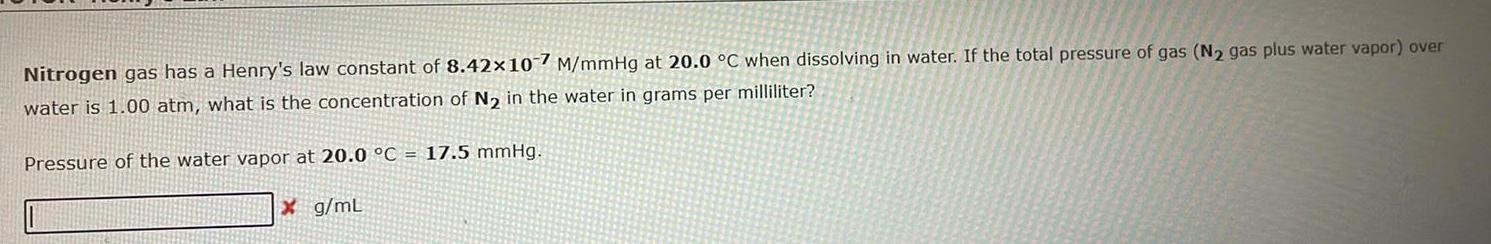Physical Chemistry
Solutions
Nitrogen gas has a Henry's law constant of 8.42x107 M/mmHg at 20.0 °C when dissolving in water. If the total pressure of gas (N₂ gas plus water vapor) over water is 1.00 atm, what is the concentration of N₂ in the water in grams per milliliter? Pressure of the water vapor at 20.0 °C = 17.5 mmHg. g/mL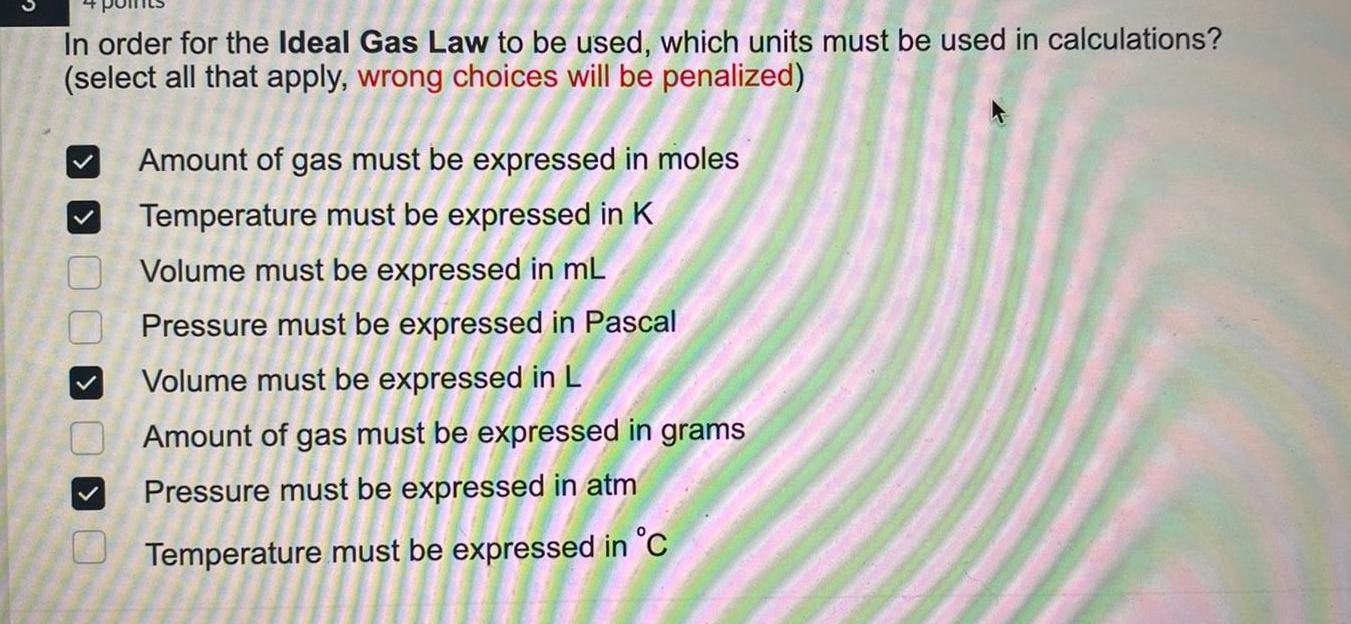Physical Chemistry
Gaseous and liquid states
In order for the Ideal Gas Law to be used, which units must be used in calculations? (select all that apply, wrong choices will be penalized) Amount of gas must be expressed in moles Temperature must be expressed in K Volume must be expressed in mL Pressure must be expressed in Pascal Volume must be expressed in L Amount of gas must be expressed in grams Pressure must be expressed in atm Temperature must be expressed in °C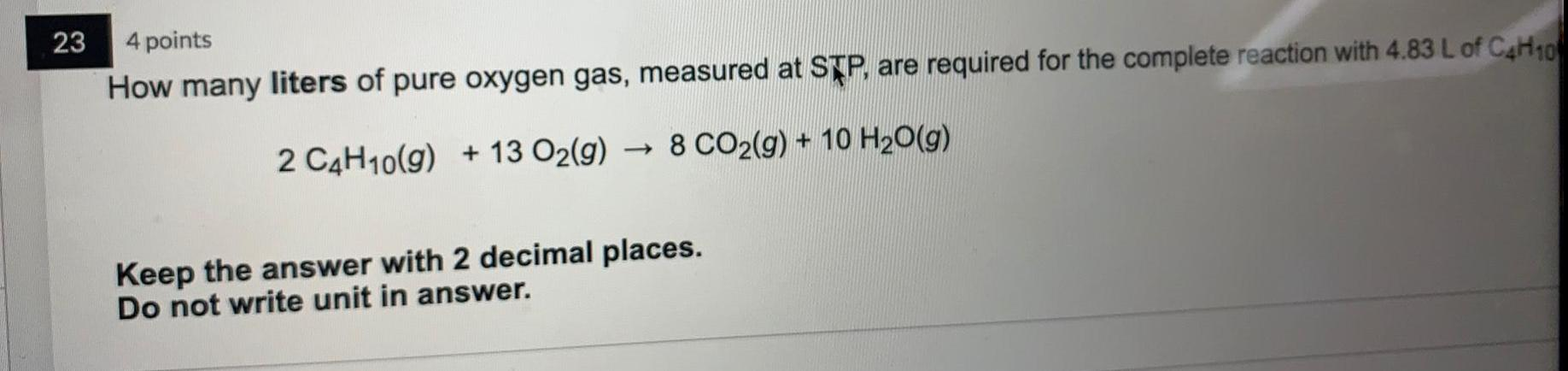Physical Chemistry
Gaseous and liquid states
How many liters of pure oxygen gas, measured at STP, are required for the complete reaction with 4.83 L of C4H10. 2 C4H10(g) + 13 O₂(g) → 8 CO₂(g) + 10 H₂O(g) Keep the answer with 2 decimal places. Do not write unit in answer.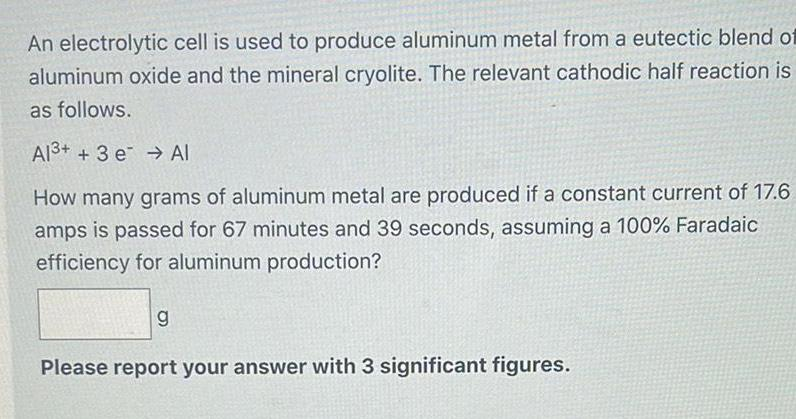Physical Chemistry
Electrochemistry
An electrolytic cell is used to produce aluminum metal from a eutectic blend of aluminum oxide and the mineral cryolite. The relevant cathodic half reaction is as follows. Al³+ + 3 e Al How many grams of aluminum metal are produced if a constant current of 17.6 amps is passed for 67 minutes and 39 seconds, assuming a 100% Faradaic efficiency for aluminum production? Please report your answer with 3 significant figures.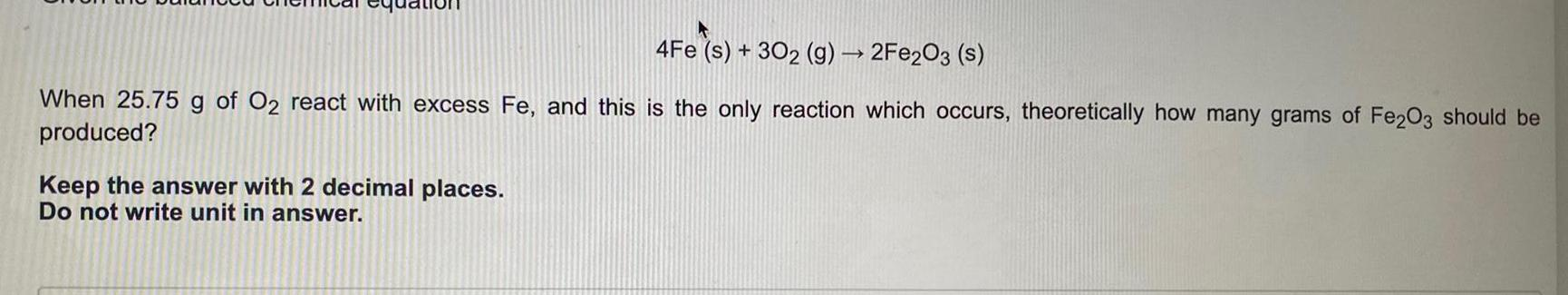Physical Chemistry
General
4Fe (s) + 302 (g) → 2Fe2O3 (s) When 25.75 g of O2 react with excess Fe, and this is the only reaction which occurs, theoretically how many grams of Fe2O3 should be produced? Keep the answer with 2 decimal places. Do not write unit in answer.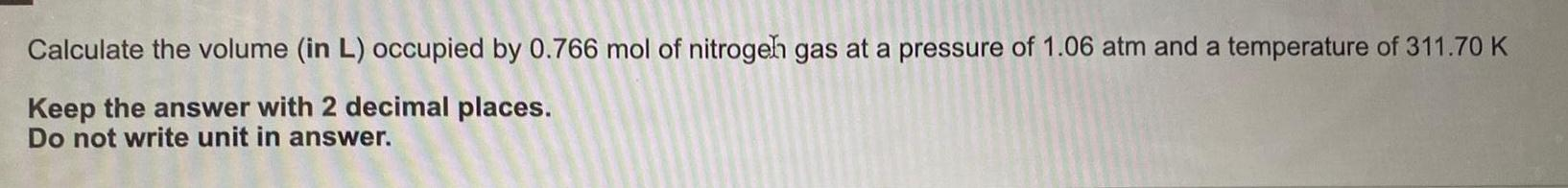Physical Chemistry
Gaseous and liquid states
Calculate the volume (in L) occupied by 0.766 mol of nitrogen gas at a pressure of 1.06 atm and a temperature of 311.70 K Keep the answer with 2 decimal places. Do not write unit in answer.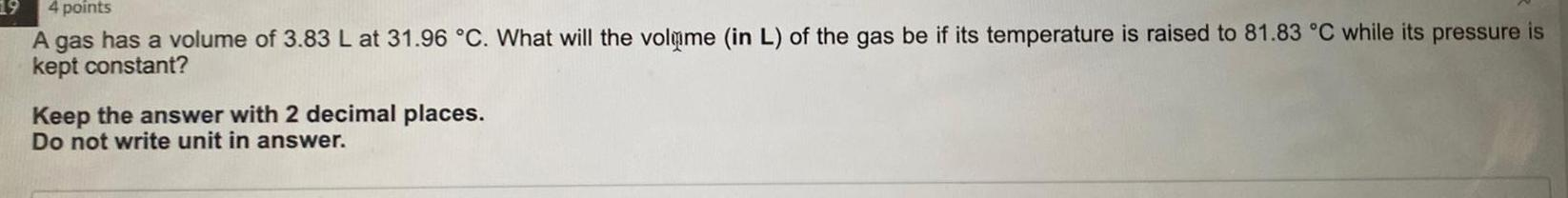Physical Chemistry
Gaseous and liquid states
A gas has a volume of 3.83 L at 31.96 °C. What will the volume (in L) of the gas be if its temperature is raised to 81.83 °C while its pressure is kept constant? Keep the answer with 2 decimal places. Do not write unit in answer.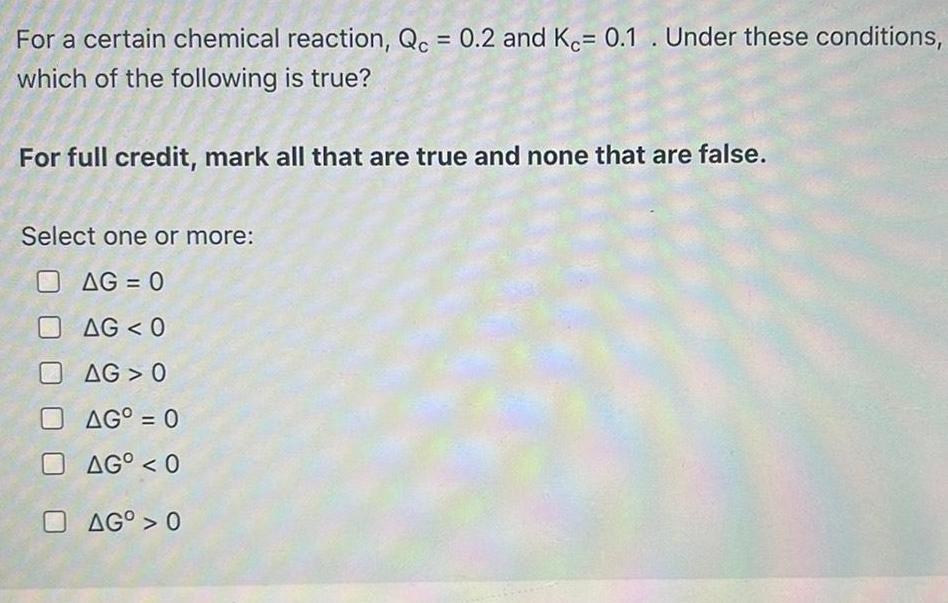Physical Chemistry
Equilibrium
For a certain chemical reaction, Qc = 0.2 and Kc= 0.1. Under these conditions, which of the following is true? For full credit, mark all that are true and none that are false. Select one or more: ΔG < 0 ΔG 0 ΔG° = 0 ΔGO < 0 ΔG° > 0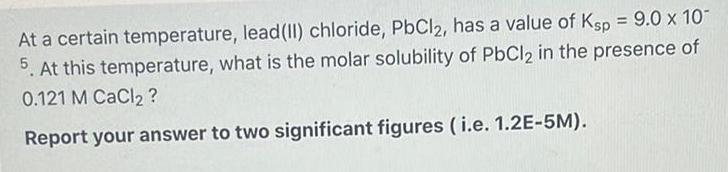Physical Chemistry
Solutions
At a certain temperature, lead (II) chloride, PbCl2, has a value of Ksp = 9.0 x 10*-5. At this temperature, what is the molar solubility of PbCl2 in the presence of 0.121 M CaCl2? Report your answer to two significant figures (i.e. 1.2E-5M).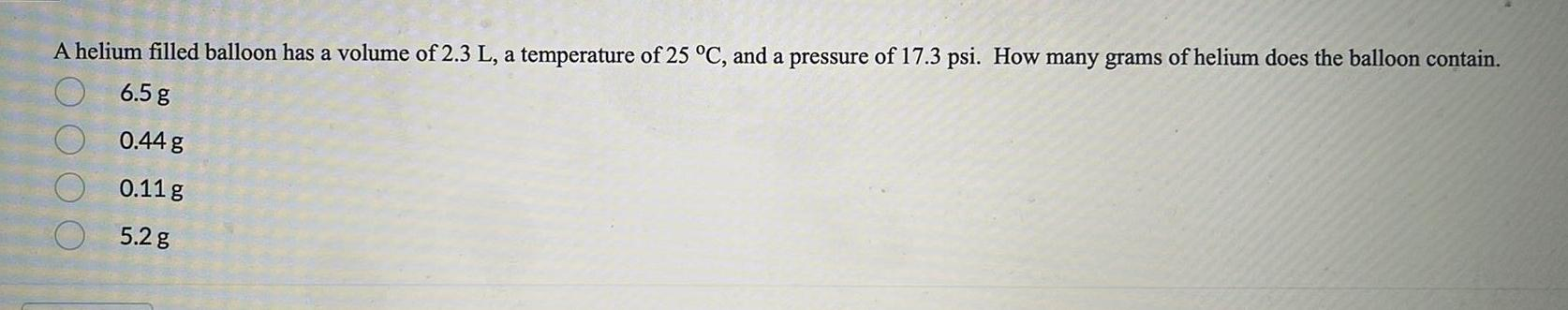Physical Chemistry
General
A helium filled balloon has a volume of 2.3 L, a temperature of 25 °C, and a pressure of 17.3 psi. How many grams of helium does the balloon contain. 6.5 g 0.44 g 0.11 g 5.2 g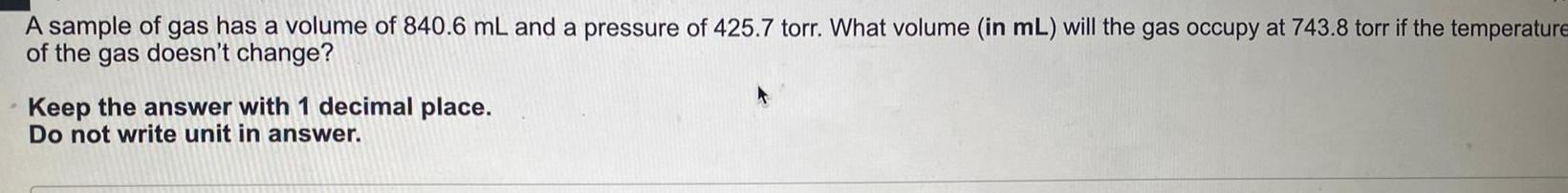Physical Chemistry
Gaseous and liquid states
A sample of gas has a volume of 840.6 mL and a pressure of 425.7 torr. What volume (in mL) will the gas occupy at 743.8 torr if the temperature of the gas doesn't change? Keep the answer with 1 decimal place. Do not write unit in answer.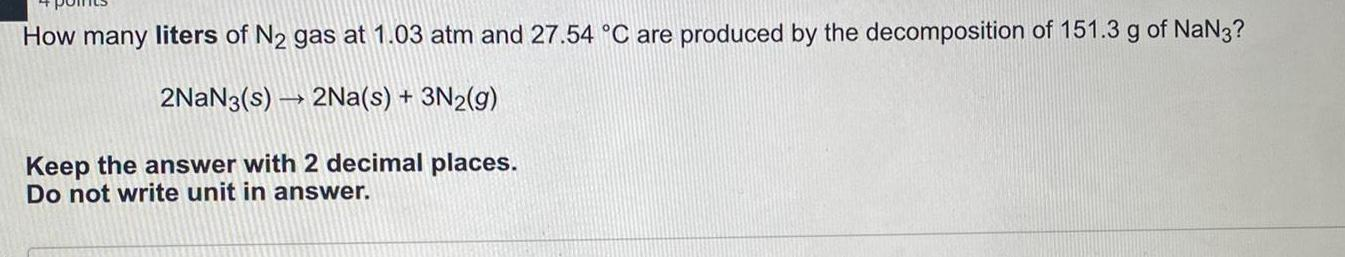Physical Chemistry
Gaseous and liquid states
How many liters of N₂ gas at 1.03 atm and 27.54 °C are produced by the decomposition of 151.3 g of NaN3? 2NaN3(s)→ 2Na(s) + 3N₂(g) Keep the answer with 2 decimal places. Do not write unit in answer.